# Functional Chaos Expansion¶

Accounting for the joint probability density function (PDF)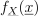of the input random vector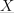, one seeks the joint PDF of the model response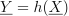. This may be achieved using Monte Carlo (MC) simulation, i.e. by evaluating the model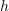at a large number of realizations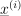ofand then by estimating the empirical distribution of the corresponding sample of model output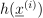. However it is well-known that the MC method requires a large number of model evaluations, i.e. a great computational cost, in order to obtain accurate results.
In fact, when using MC simulation, each model run is performed independently. Thus, whereas it is expected that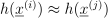if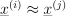, the model is evaluated twice without accounting for this information. In other words, the functional dependence betweenand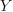is lost.
A possible solution to overcome this problem and thereby to reduce the computational cost of MC simulation is to represent the random responsein a suitable functional space, such as the Hilbert space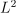of square-integrable functions with respect to the PDF. Precisely, an expansion of the model response onto an orthonormal basis ofis of interest.
The principles of the building of a (infinite numerable) basis of this space, i.e. the PC basis, are described in the sequel.
Principle of the functional chaos expansion
Consider a modeldepending on a set of random variables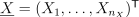. We call functional chaos expansion the class of spectral methods which gathers all types of response surface that can be seen as a projection of the physical model in an orthonormal basis. This class of methods uses the Hilbertian space (square-integrable space:) to construct the response surface.
Assuming that the physical model has a finite second order measure (i.e.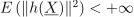), it may be uniquely represented as a converging series onto an orthonormal basis as follows: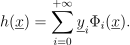where the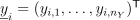’s are deterministic vectors that fully characterize the random vector, and the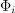’s are given basis functions (e.g. orthonormal polynomials, wavelets).

The orthonormality property of the functional chaos basis reads: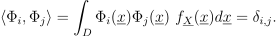where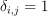if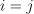and 0 otherwise. The metamodel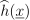is represented by a finite subset of coefficients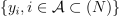in a truncated basis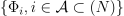as follows: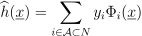As an example of this type of expansion, one can mention responses by wavelet expansion, polynomial chaos expansion, etc.